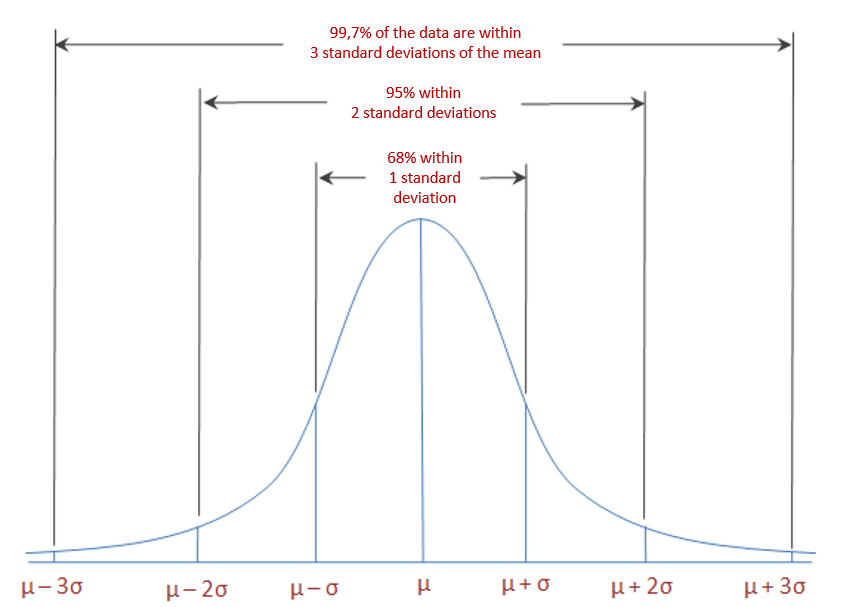# 5.1.3 Summarizing Data Numerically

### Measures of Central Tendency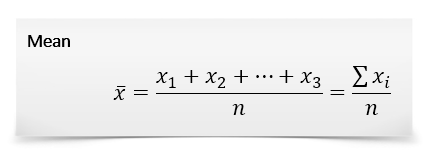• It works well for lists that are simply combined (added) together.
• Easy to calculate: just add and divide.
• It’s intuitive — it’s the number “in the middle”, pulled up by large values and brought down by smaller ones.

• The average can be skewed by outliers — it doesn’t deal well with wildly varying samples.
• The average of 100, 200 and -300 is 0, which is misleading.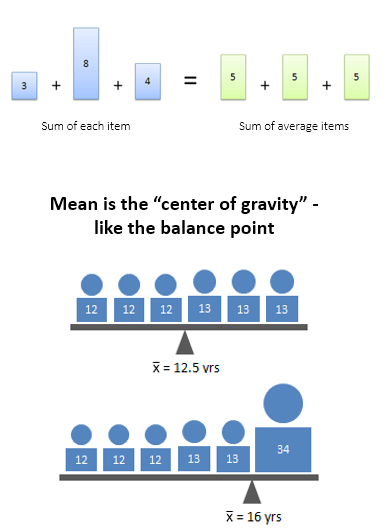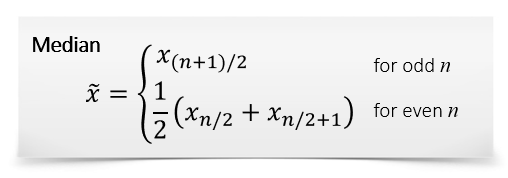• Handles outliers well — often the most accurate representation of a group
• Splits data into two groups, each with the same number of items

• Can be harder to calculate: you need to sort the list first
• Not as well-known; when you say “median”, people may think you mean “average”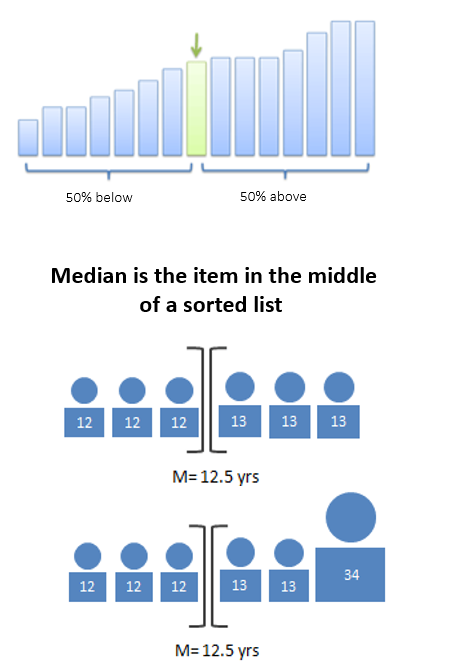Mode

• Works well for exclusive voting situations (this choice or that one; no compromise), i.e., for nominal data
• Gives a choice that the most people wanted (whereas the average can give a choice that nobody wanted).
• Simple to understand

• Requires more effort to compute (have to tally up the votes)
• “Winner takes all” — there’s no middle path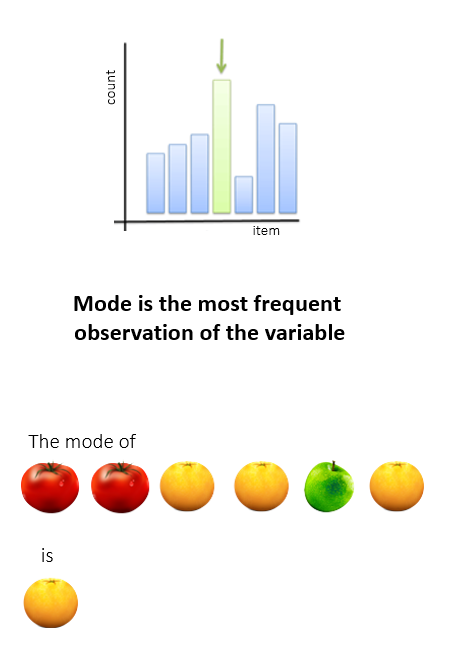### Measures of Central Tendency: Using Mean and Median to Identify the Distribution Shape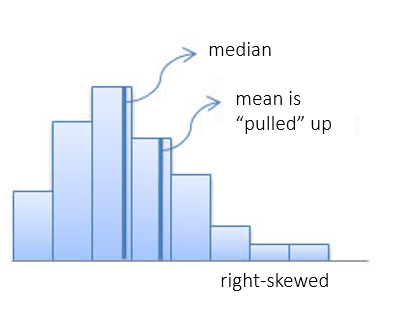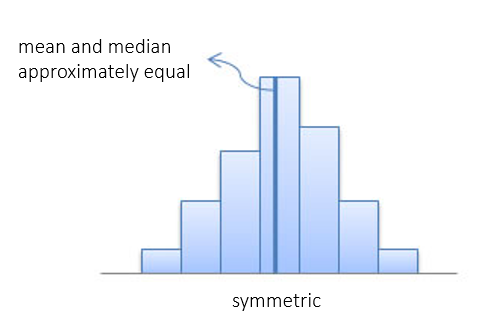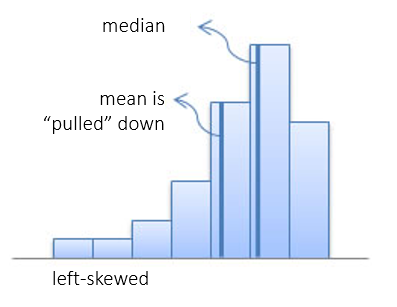### Measures of Dispersion

Variance is the average of the squared distance form the mean

Population Variance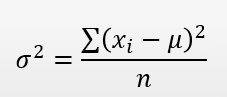Sample Variance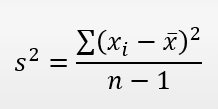Why Variance?

Mean acts as a balancing point. Hence, the average difference from the mean will equal zero.

When calculating variance, all differences are squared, so that negative differences do not compensate positive differences.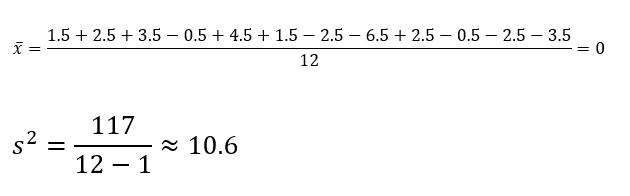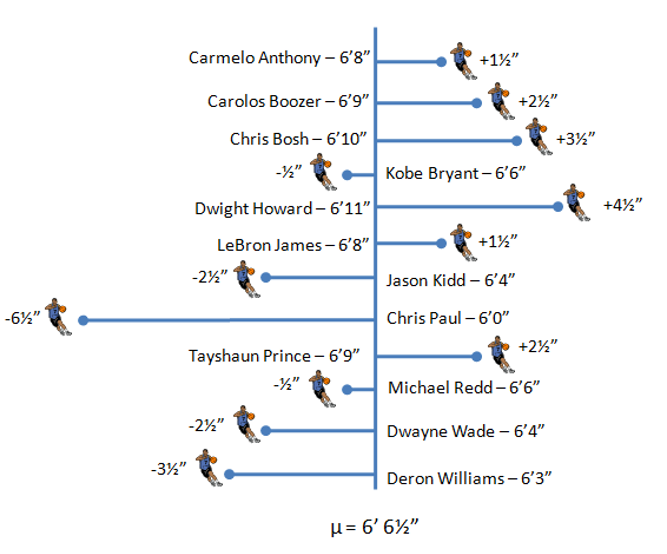Standard Deviation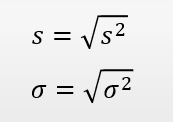Standard Deviation keeps the units of the original measure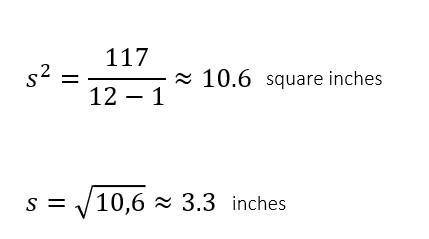Which data set has a higher standard deviation?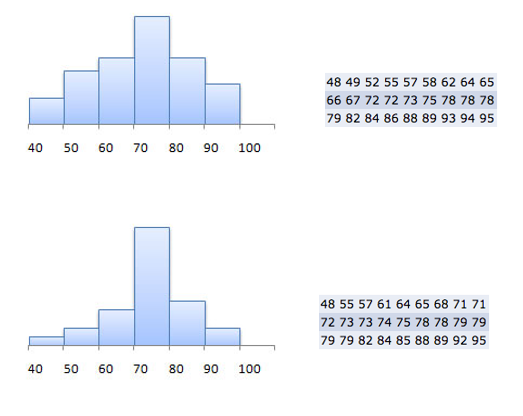### Relationship between the Standard Deviation and the Shape of the Normal Distribution